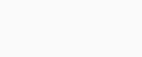# Python program to calculate the Standard Deviation

In this article, we are going to understand about the Standard Deviation and how it is calculated in Python. Before the calculation of Standard Deviation, we need to understand what does it mean. Standard Deviation is the measure of spreads of data from the mean value of that data.

If the standard deviation has low value then it indicates that the data are less spread from there mean value and if it has high value then it indicates that the data is more spread out from their mean value.

## Calculation of Standard Deviation in Python

Standard deviation is calculated by two ways in Python, one way of calculation is by using the formula and another way of the calculation is by the use of statistics or numpy module.

The Standard Deviation is calculated by the formula given below:-

``

Where N = number of observations, X1, X2,………, XN = observed values in sample data and Xbar = mean of the total observations.

Example 1:- Calculation of standard deviation using the formula

```observation = [1,5,4,2,0]
sum=0
for i in range(len(observation)):
sum+=observation[i]
mean_of_observations = sum/len(observation)

sum_of_squared_deviation = 0
for i in range(len(observation)):
sum_of_squared_deviation+=(observation[i]- mean_of_observations)**2
Standard_Deviation = ((sum_of_squared_deviation)/len(observation))**0.5
print("Standard Deviation of sample is ",Standard_Deviation)```

Output:-

`Standard Deviation of sample is  1.854723699099141`

In the above example, we first calculated the mean of the given observation and then we calculated the sum of the squared deviation by adding the square of the difference of each observation from the mean of the observation.

Then we calculated the standard deviation by taking the square root of the division of the sum of squared deviation and number of observations.

Example 2:- Calculation of standard deviation using the numpy module

```import numpy as np

# creating a simple data - set
sample = np.array([1,5,4,2,0])

# Prints standard deviation
print("Standard Deviation of sample is % s "% (np.std(sample)))```

Output:-

`Standard Deviation of sample is 1.8547236991`

In this example, we imported the numpy module and then we created a numpy array. Then we calculated the standard deviation by using the function np.std(), by this method we got the required standard deviation.

### One response to “Python program to calculate the Standard Deviation”

1. Cameron says:

What would i have to change in the calculation of stantard devaiation using the formula if i was to use 4 data points eg: 1.97, 2.05 , 2.08 , 2.45 . I have tried substituting the numbers in the observation and changing the sum to the sum of my data but i dont know if thats what im supposed to do